# What is the real meaning of bending stresses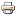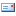This mechanics script shows how the load on bending can be calculated. More precisely, this is about the calculation of the bending stress of a beam that is loaded with a transverse force (differentiate between pure and transverse force-free bending). To understand the bending load on a beam, here are some key basics:

### Basics of bending stress

If long, thin components such as shafts, rods or beams are loaded with a bending moment across the component axis, both tensile and compressive stresses arise, which ultimately result in deflection. If you want to calculate this bend, the following understanding is important:

In the area of ​​the tensile forces, the affected component is stretched, whereas it is compressed in the area in which the compressive forces act. The layer in the middle between these two areas is the so-called neutral fiber. Neither elongation nor compression takes place along this line. According to the definition, the tension emanating from the neutral fiber increases steadily in the direction of the outer component edge. As a result, the strongest stresses occur on the outer edge of the component.

#####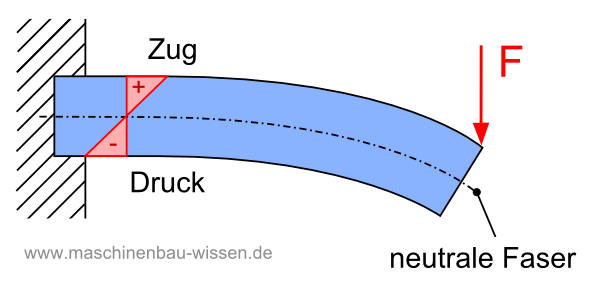Figure: Bending load on a beam with tensile and compressive stresses

You can find more about beam bending in the main section:
Beam bending script

### Example 1 - bending stress on a component

The rod, shaft or beam is subjected to bending as a result of the force F, whereby the originally straight rod axis bends. As can be seen, both tensile and compressive stresses arise within the cross-section. In order to clearly illustrate this fact, the extent of the deflection has been shown greatly exaggerated in the sketch.

Here is a hint that this example is a so-called straight bend and a shear force bend. Read more about the different types of bend in this script:

Types of bend

#####Figure: Bending load on a beam with tensile and compressive stresses

The neutral fiber (dash-dot representation) is completely stress-free, while the strongest stresses can be seen at the edges of the rod. The following applies to symmetrical cross-sections: The tensile and compressive stresses are always distributed linearly, i. H. evenly over the entire cross-section of the component.

### Calculate bending stress - formula

The following formula is used to calculate the bending stress: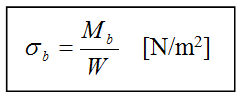σb - bending stress
M.b - Bending moment
W - section modulus

From this formula it can be seen that the bending stress σb is dependent

• from the bending moment Mb and
• from section modulus W.

How to calculate these two quantities, read in the following.

#### The bending moment Mb to calculate

In the following, only formulas for simple load cases are given. In order to avoid confusing and complicated calculations, the bending moment is determined for all other cases using formulas and tables that can be found in books of tables.

In practice, the following load cases can be found for bending moments Mb with a single force acting particularly often:

• girder clamped on one side
• Beam that rests on two supports
#####Two typical load cases for beam bending by means of shear force

For the beam clamped on one side, as shown in example 1, the following applies: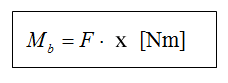M.b - Bending moment
F - force (lateral force)
x - distance (of the force F from the fixed bearing of the beam)

#### The axial section modulus W

The axial section modulus is a measure of the resistance to deflection. That is why it is often referred to as the bending resistance moment. Only the geometry of the component cross-sectional area under consideration is decisive for the size of the section modulus.

The definition of the exact position of the neutral fiber within the cross-section is a basic requirement for calculating the section modulus. The neutral fiber runs exactly through the center of gravity of the cross-section. Starting from this line, the greatest possible distance to the outer edge (edge ​​fiber) can then be determined, where the highest component loads or the greatest stresses are to be expected.

The moment of resistance is calculated as the quotient of the area moment of inertia and the distance (aMax), which represents the dimension from the tension-free neutral fiber to the outer edge (edge ​​fiber).W - axial section modulus
I - axial moment of area 2nd degree (also area moment of inertia)
aMax: greatest distance between the edge fiber and the neutral fiber

#### The area moment of inertia

The area moment of inertia (also second area moment) - more precisely the axial area moment of inertia - defines the resistance of a component to bending. The calculation is made as a derivation from the cross-sectional geometry of the rod, beam, shaft or similar. The area moment of inertia is usually specified in the SI unit m4.
It is best to use suitable tables to calculate the geometrical moment of inertia, as the independent derivation is relatively time-consuming.

In the picture below you can see two examples for the calculation of the geometrical moment of inertia and the bending resistance moment.

#####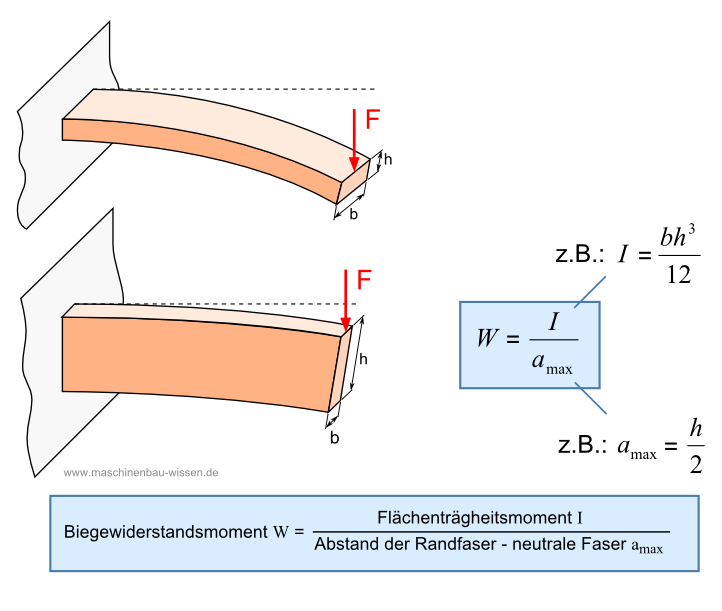With the calculated values ​​- bending moment, area moment of inertia and resistance moment - you can now go to the formula shown at the beginning and calculate the bending stress.

More scripts with the basics of the area moment of inertia can be found here:

### Example 2 - Beam rests on two supports

The second example shows the second typical case for a bending load. Here you can calculate the bend in a relatively similar way.

#####Beam loaded with central transverse force

The force F acts centrally on the beam resting on two supports. The bending moment is obtained by viewing the bending cross-section from the side, as shown in the picture. Both the force F / 2 and the distance x / 2 can be seen. The bending moment is now calculated as follows: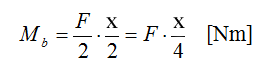M.b - Bending moment
F - force (lateral force)
x - distance (of the force F from the fixed bearing of the beam)

The section modulus and area moment of inertia are independent of the bending moment and can be calculated in the same way as in the previous example. The bending stress can then also be determined using the bending moment.

Annotation:
The bending stresses and bending moments calculated here are the maximum values ​​that occur in each case.

#####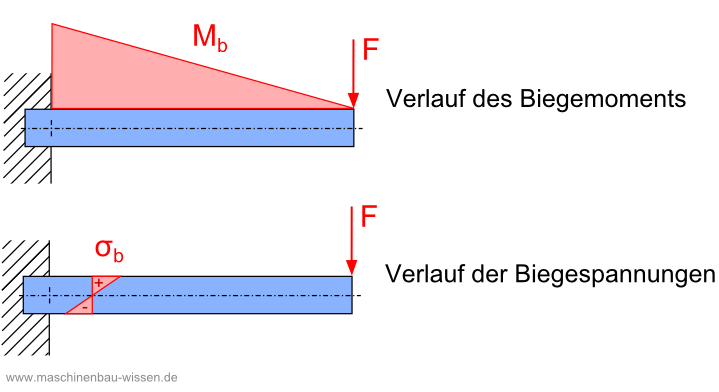Different values ​​for bending moment and bending stress

Generally speaking, the amount of the bending moment depends on the measuring point on the beam (usually declared as the x-coordinate).
The level of the bending stress depends on the level of the bending moment and thus in turn depends on the measuring point on the beam. If one looks at the inside of the beam, the bending stress is highest in the edge fiber with the greatest distance to the neutral fiber. The bending stress is therefore also dependent on the point within the cross-section of the beam at which the measurement is carried out (usually the z-coordinate).